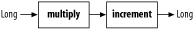# 4.11. Creating a Chain of Transformations

## Problem

You have a series of transformations and you need to chain them together, passing the output of one stage to the input of the next.

## Solution

Create multiple implementations of `Transformer`, and chain them together with `ChainedTransformer`. A `ChainedTransformer` takes an array of `Transformer` objects, passing the output of each `Transformer` to the next `Transformer` in the chain. The following example demonstrates a `ChainedTransformer` with two `Transformer` stages. The first stage, `multiply`, multiplies a number by 100, and the second stage, `increment`, adds one to the result from the first stage:

```import org.apache.commons.collections.Transformer;
import org.apache.commons.collections.functors.ChainedTransformer;

Transformer multiply = new Transformer( ) {
public Object transform(Object input) {
Long number = (Long) input;
return( new Long( number.longValue( ) * 100 ) );
}
}

Transformer increment = new Transformer( ) {
public Object transform(Object input) {
Long number = (Long) input;
return( new Long( number.longValue( ) + 1 ) );
}
}

Transformer[] chainElements = new Transformer[] { multiply, increment };
Transformer chain = new ChainedTransformer( chainElements );

Long original = new Long( 34 );
Long result = chain.transform( original );

System.out.println( "Original: " + original );
System.out.println( "Result: " + result );```

The `Transformer` chain takes the `Long` instance `original` and transforms it into a result:

```Original: 34
Result: 3401```

## Discussion

Since a `Transformer` leaves the input parameter passed to `transform( )` intact, this two-stage `ChainedTransformer` creates a new instance of `Long` for each stage in the `ChainedTransformer`. A `Long` is passed to the first stage, `multiply`, which transforms 34 to 3400. The result from the first stage, 3400, is then passed to the second stage, `increment`, which produces the final `Long` result, 3401. A real example would involve more complex implementations of `Transformer`, but this simple example demonstrates the mechanics of creating a simple pipeline of transformations, one leading to another. Figure 4-4 illustrates the simple structure of this two-staged `ChainedTransformer`.Figure 4-4. A ChainedTransformer with two Transformers

Jakarta Commons Functor in the Commons Sandbox introduces a `UnaryFunction` interface that provides an interface equivalent to `Transformer`, and multiple `UnaryFunction` instances can be chained together using the `CompositeUnaryFunction` class. For more information about `CompositeUnaryFunction`, see the Commons Functor page at http://jakarta.apache.org/commons/sandbox/functor.